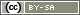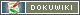[[Scale]]
This translation is older than the original page and might be outdated. See what has changed.

# Scale

SoSci Survey provides different question types for the realisation of rating-scales. In the following, we will look at rating-scales with a item text (for example a statement) on one side and several response levels next to it (or below) to assess the item.

picture is still missing «

Depending on whether you want to label only the extreme values ​​of the scale or whether you want to label each individual value, please use the question type “scale (extrema labeld)” or “scale (interim values labeled). You can also change the question type later.

Tip: Under DisplayArrangement, select whether the scale points should be displayed next to the item text or below.

## Encoding the response options

The selection options on a scale automatically receive the codes 1 (minimum) to k (maximum). k is the number of scale levels. Thus, e.g. 1 to 5 for a 5 scale.

### Inverting items

You can invert the encoding of single items (reversed coding) so that a selection of the minimum (for example, “does not apply at all”) is not code 1 but code 5 (on a 5-level scale). To do this, either select a checkmark to the right of the item text (⮀) in the Item quick-entry or select the item on the left in the navigation (Question catalog) and activate the option Invert answer codes for this item.

### Recode items

If you need a different encoding (for example, 0..4 instead of 1..5), you have two options: You can recode the items classically during evaluation - or you can recode them into new variables within the questionnaire.

For this, you first need a question Internal Variables with as many variables as your scale has items. The easiest way to do this is by duplicating the question with the scale and then changing the question type of the copy to “Internal Variables”.

Then select with PHP-Code and the function `value()` the subscriber's response in the scale, make the desired transcoding, and save the result using `put()` to one of the internal variables.

Important: The PHP code may not be on the same page as the scale, but on the next page (e.g., at the beginning of the next page). For details, please refer to the notes on the function `value()`.

#### Example 1

The following PHP code encodes all items of the “SC01” scale so that the encoding 1..5 is transformed to 0..4. The recoded values ​​are stored in the internal variables of “IV01”.

```\$input = 'SC01';
\$output = 'IV01';
// Create a list of all items
\$items = getItems(\$input, 'all');
// Go through all items
foreach (\$items as \$item) {
// id() constructs an item identifier (SC01_01) from a question identifier (SC01) and the item number (e.g., 1)
// value() has the answer code expelled
\$orgCode = value(id(\$input, \$item));
// Subtract from 1
\$newCode = \$orgCode - 1;
// put() stores the value in an internal variable
put(id(\$output, \$item), \$newCode);
}```

Important: The question “internal variables”, in example IV01, must be created manually in the question catalog (see above).

Important: The above code also transforms error codes, e.g. is -1 for the selection of a residual option (“do not know”) -2. Code -9 (no answer) would be -10.

#### Example 2

The following PHP code requires an individual recoding for each item. This is initially defined in an array. The identifier of the item is used as the key to the array, that is, e.g. 2 for “SC01_02”

Within the array, further arrays are defined (in the example, one per line), which specifies the exact recoding. In the second item, e.g. the value 1 becomes 5, the values ​​2 and 3 become 2, the value 4 becomes 1 and the 5 becomes the 0.

```\$input = 'SC01';  // ID of the scale question
\$output = 'IV01'; // ID of the internal variable

\$recode = array(
1 => array(1 => 2, 2 => 4, 3 => 6, 4 => 8, 5 => 10),
2 => array(1 => 5, 2 => 2, 3 => 2, 4 => 1, 5 => 0),
3 => array(1 => 1, 2 => 1, 3 => 2, 4 => 2, 5 => 4),
4 => array(1 => 0, 2 => 2, 3 => 2, 4 => 1, 5 => 0)
);

foreach (\$recode as \$item => \$codes) {
// Retrieve the response code from the scale
\$response = value(id(\$input, \$item));
// Recode only if this value has been defined (otherwise save the error code -8)
if (isset(\$codes[\$response])) {
put(id(\$output, \$item), \$codes[\$response]);
} else {
put(id(\$output, \$item), -8);
}
}```

## Different inscriptions

The response options on a scale are the same for all items, e.g. from “does not apply at all” until “fully meets”. If your items require different response options, first check whether one of the following question types is suitable:

If not, create several scale questions and display them directly in the questionnaire Concatenating Questions